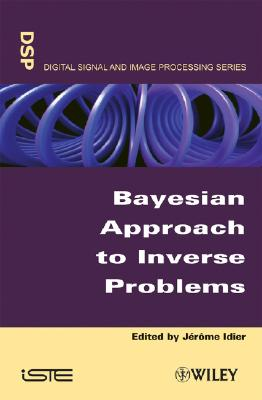### Bayesian Approach to Inverse Problems (Hardcover)

By Jerome Idier (Editor)

Wiley-Iste, 9781848210325, 381pp.

Publication Date: June 1, 2008

List Price: 206.95*
* Individual store prices may vary.

#### Description

Many scientific, medical or engineering problems raise the issue of recovering some physical quantities from indirect measurements; for instance, detecting or quantifying flaws or cracks within a material from acoustic or electromagnetic measurements at its surface is an essential problem of non-destructive evaluation. The concept of inverse problems precisely originates from the idea of inverting the laws of physics to recover a quantity of interest from measurable data.

Unfortunately, most inverse problems are ill-posed, which means that precise and stable solutions are not easy to devise. Regularization is the key concept to solve inverse problems.

The goal of this book is to deal with inverse problems and regularized solutions using the Bayesian statistical tools, with a particular view to signal and image estimation.

The first three chapters bring the theoretical notions that make it possible to cast inverse problems within a mathematical framework. The next three chapters address the fundamental inverse problem of deconvolution in a comprehensive manner. Chapters 7 and 8 deal with advanced statistical questions linked to image estimation. In the last five chapters, the main tools introduced in the previous chapters are put into a practical context in important applicative areas, such as astronomy or medical imaging.